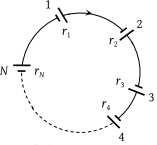A group of N cells whose emf varies directly with the internal resistance as per the equation EN = 1.5 rN are connected as shown in the figure below. The current I in the circuit is(1) 0.51 amp

(2) 5.1 amp

(3) 0.15 amp

(4) 1.5 amp

(4) $i=\frac{{E}_{1}+{E}_{2}+{E}_{3}+.....+{E}_{n}}{\left({r}_{1}+{r}_{2}+{r}_{3}+.......+{r}_{n}\right)}$

$=\frac{1.5\left({r}_{1}+{r}_{2}+{r}_{3}+......+{r}_{n}\right)}{\left({r}_{1}+{r}_{2}+{r}_{3}+.....+{r}_{n}\right)}=1.5A$.

Difficulty Level:

• 25%
• 15%
• 20%
• 40%